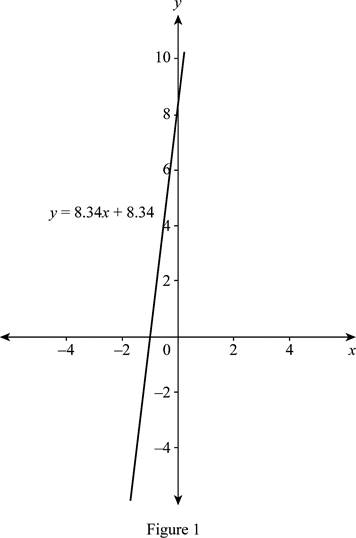# The slope of the graph c and interpret it.### Single Variable Calculus: Concepts...

4th Edition
James Stewart
Publisher: Cengage Learning
ISBN: 9781337687805### Single Variable Calculus: Concepts...

4th Edition
James Stewart
Publisher: Cengage Learning
ISBN: 9781337687805

#### Solutions

Chapter 1.2, Problem 11E

(a)

To determine

## To find: The slope of the graph c and interpret it.

Expert Solution

The slope of the function c is 8.34 mg, which represents the rate of change of dosage.

### Explanation of Solution

Rewrite the given equation as c=0.0417aD+0.0417 (1)

In the equation, c=0.0417aD+0.0417 , the slope is 0.0417D and the c-intercept is 0.0.417.

Given that the dosage of the adult (D) is 200 mg.

Substitute D=200 in the equation (1) and obtain the appropriate dosage of a child as follows.

c=0.0417a(200)+0.0417=8.34a+8.34

Thus, c=8.34a+8.34 (2)

Therefore, the slope is 8.341, which represents the average rate of change of the dosage in mg. The dosage is calculated for a one year old child.

The graph of c is shown below in Figure 1.From Figure 1, it is observed that the graph of c is a straight line as the function is linear.

(b)

To determine

### To find: The dosage for a newborn.

Expert Solution

The dosage for a newborn is 8.34 mg.

### Explanation of Solution

Since age (a) of a newborn child is 0, substitute a=0 in equation (2) and obtain the dosage as follows.

c=8.34a+8.34=8.34(0)+8.34=8.34

Therefore, for a newborn child the advisable appropriate dosage is 8.34 mg.

### Have a homework question?

Subscribe to bartleby learn! Ask subject matter experts 30 homework questions each month. Plus, you’ll have access to millions of step-by-step textbook answers!